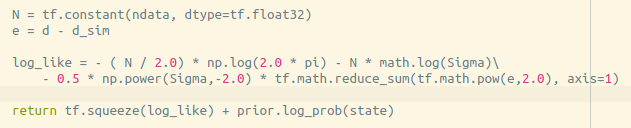Building a p(theta|d) model for the NeuTra rountine

Hello Pyro community!
Thank you so much for very nice documentation and examples, they are very helpful!
I am sorry in advance if the answer to my question is obvious, I am a bit confused with some of the features of Pyro.
I am trying to use the NeuTra routine of Hoffman et al. 2019 to approximate a posterior distribution p(theta|d). In the TensorFlow implementation I simply calculated the posterior log probability for theta samples drawn out of the variational density q(theta) as follows:but in Pyro I got a bit confused when I went over the “Example: Neural MCMC with NeuTraReparam”.
If I understand it correctly, in the pyro example a distribution object is provided (analytical solution) and from which you can sample and automatically calculate the log probability.
In my case I don’t know how to represent my posterior as an object (maybe there is a way?) to sample from, but only provide the log posterior probability for specific theta that is drawn out of q(theta) by calculating the log likelihood p(d|theta) and multiply it by the prior of theta p(theta).
How can I implement a model for this case?

Hi @dodi56, I think you can just create a distribution with dummy sample method like in this neutra example.

Hi @fehiepsi, thanks for the response!
So the sample() method here would only be for shape indication?
Another question:
If I would like to use batches for training the neutra, should I include the batch shape in the sample() method, or just use
with pyro.plate(‘batch’, batch_size):
pyro.sample(“x”, Posterior())

or both?

Thanks!

For training in batches, you can use subsample in the model likelihood. No need to worry about the guide. You can find more examples here for how to use subsample in Pyro. This example seems to be most relevant to your model where coefs is theta and obs is your d.